螢幕快照

•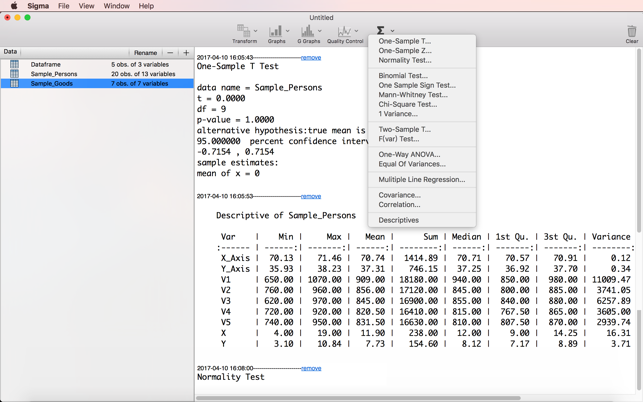•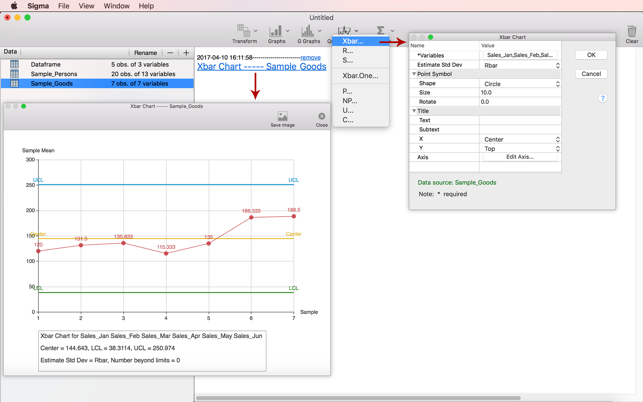•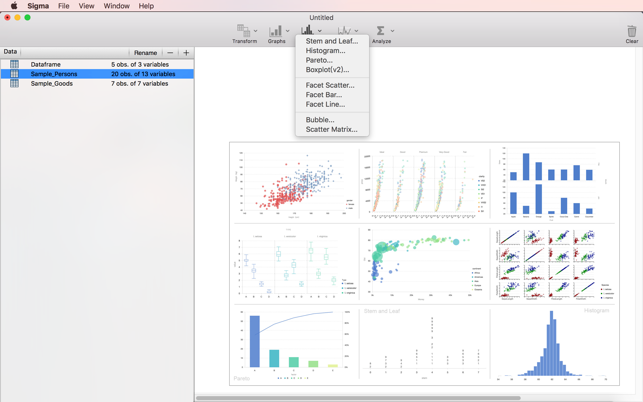•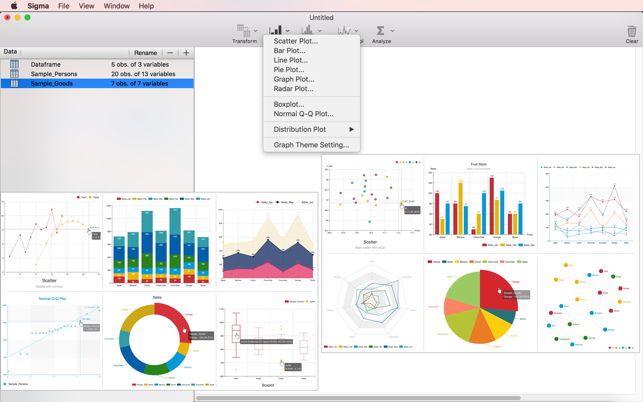•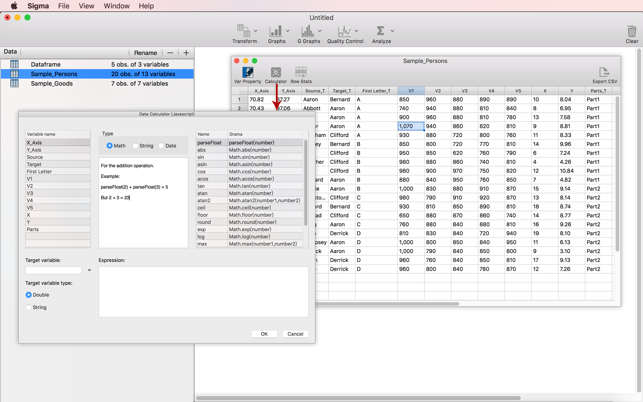簡介

Sigma is a powerful statistical software everyone can use. Analyze your data and plot it.More than 15 H-Test method,18 types of probability distribution.

+ Probability Distributions
Normal, Chi Square, F, T, Uniform, Poisson, Geometric, Negative Binomial, Hypergeometric, Beta, Cauchy, Exponential, Gamma, LogNormal, Logistic,
Wilcoxon, Weibull, Wilcoxon Rank Sum Statistic.

+ Plots
- Scatter Plot
- Line Plot
- Pie Plot
- Bar Plot
- Box Plot
- Graph Plot
- Normal And Chisquare Distribution Plot

+ Special Plots
- Stem And Leaf
- Pareto
- Histogram
- Facet Scatter
- Facet Bar
- Facet Line
- Facet Pie
- Facet Area
- Jitter Point
- Nightingale Rose
- Bubble
- Scatter Matrix

+ Hypothesis Tests
- Normality Test (Anderson Darling, Jarque-Bera, Shapiro- Wilk)
- One-Sample T Test
- Two-Sample T Test
- One-Sample Z Test
- Chi-Square Test
- Mann-Whitney U Test
- One Sample Sign Test
- One Variance
- F(Var) Test
- Binomial Test
- Kolmogorov–Smirnov Test
- One-Way ANOVA
- Two-Way ANOVA
- Levene Test
- Bartlett Test
- Flinger Test
- Multiple Line Regression
- Binary Logistic Regression
- Correlation Matrix And Correlation Test(Pearson and Spearman)
- Covariation Matrix
- Two Sample Z Test
- Two Proportion Z Test
- Two Sample Sign Test

+ Control Charts
- Xbar Chart
- R Chart
- S Chart
- Xbar.One Chart
- P Chart
- NP Chart
- U Chart
- C Chart

評分與評論

5.0（滿分 5 分）
1 則評分

1 則評分

Wmlitt

Good statistics app

The app keeps getting better with each new update.

資訊

YUANQUAN LI

11.9 MB

OS X 10.10 or later, 64-bit processor

English

Rated 4+
•